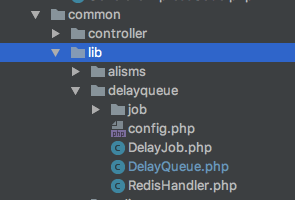# php取得当前时间函数

+关注继续查看

php取得当前时间函数文章提供了php的几种获取当前时间的函数，date,time等哦，同时告诉我如何解决时区问题哦。

php获取当前时间

<?php echo $showtime=date("Y-m-d H:i:s");?> //2010-08-29 21:25:26 //Y是4位的年，H是24小时制 -------------------------------------------------------------------------- php教程取得当前时间函数 文章提供了php的几种获取当前时间的函数，date,time等哦，同时告诉我如何解决时区问题哦。 方法一date函数 echo date(‘y-m-d h:i:s’,time()); //2010-08-29 11:25:26 方法二 time函数$time = time();

echo date("y-m-d",$time) //2010-08-29 方法三$_server['server_time']

echo strftime ("%hh%m %a %d %b" ,time());

18h24 sunday 21 may

------------------------------------------------------

php获取当前时间

<?php echo $showtime=date("Y-m-d H:i:s");?> 显示的格式: 年-月-日 小时:分钟:秒 相关时间参数:(注意大写和小写的区分) a - "am" 或是 "pm" A - "AM" 或是 "PM" d - 几日，二位数字，若不足二位则前面补零; 如: "01" 至 "31" D - 星期几，三个英文字母; 如: "Fri" F - 月份，英文全名; 如: "January" h - 12 小时制的小时; 如: "01" 至 "12" H - 24 小时制的小时; 如: "00" 至 "23" g - 12 小时制的小时，不足二位不补零; 如: "1" 至 12" G - 24 小时制的小时，不足二位不补零; 如: "0" 至 "23" i - 分钟; 如: "00" 至 "59" j - 几日，二位数字，若不足二位不补零; 如: "1" 至 "31" l - 星期几，英文全名; 如: "Friday" m - 月份，二位数字，若不足二位则在前面补零; 如: "01" 至 "12" n - 月份，二位数字，若不足二位则不补零; 如: "1" 至 "12" M - 月份，三个英文字母; 如: "Jan" s - 秒; 如: "00" 至 "59" S - 字尾加英文序数，二个英文字母; 如: "th"，"nd" t - 指定月份的天数; 如: "28" 至 "31" U - 总秒数 w - 数字型的星期几，如: "0" (星期日) 至 "6" (星期六) Y - 年，四位数字; 如: "1999" y - 年，二位数字; 如: "99" z - 一年中的第几天; 如: "0" 至 "365" 可以自由设定显示的内容,连接符号或是显示位置,例如 date("m-d H") 或者date("dmY");?>等 php中的日期处理 加入时间：2004-12-18 17:35:22 大小:12 KB 阅读次数:1405 PHP中的日期处理 转贴：xiaxia 日期：2004-05-26 人气：9 我正打算用PHP编写一种帮助处理系统。我发现我必须知道处理完最后一位客户的问题后已经过去了多长时间？当我过去用ASP时解决这个问题相当简 单，ASP有相应的函数DateDiff可以给出两个日期间间隔多少月、多少天和多少秒。当我搜寻完PHP手册后我发现PHP并没有类似的函数。 本文包含以下内容： 1、 得到目前的日期和时间－我们有多少种方式？ 2、 改变日期显示的方式－日期和时间的显示形式 3、 转换现在的日期为Unix的时间戳值 4、 改变日期 a. 增加时间 b. 减去时间 c. 找出两日期之间的间隔 5、 为PHP添加DateAdd函数 6、 为PHP添加DateDiff函数 **得到目前的日期和时间 在Unix中，时间的表示方式为计算从1970年1月1日零时起所过去的秒数，这称为UNIX 时间戳(Unix Epoch)。 如果我们有这样一段的代码： echo time(); 将返回值958905820 而此时的时间为2000年5月21日12时43分。 你也许会说这相当不错。当这对我毫无帮助，或者只有一点帮助。在PHP中，对日期处理的函数都必须用到由time()返回的时间戳值。同时，由于PHP在 Unix和Windows系统中均使用同样的时间戳值，这就允许你不需要修改代码即可在不同的系统间移植。另外的一个好处是time()函数返回的是一个 整数，你可以将其作为整数字段或文本字段存入数据库，而不必使用特别的日期/时间字段。 你已经基本了解了Unix的时间戳值，现在让我们来展示它的实际用途。 改变日期显示的方式－日期和时间的显示形式 PHP提供两个办法来将Unix的时间戳值转换成为有用的数据。第一个是date()函数。这个函数有两个参数－第一个字符串用于设定你所希望返回的格式，第二个为Unix的时间戳值。 格式化字符串通过一些简单的特殊格式化字符来显示你所希望看到的格式的日期和时间。假设你希望日期以这样的格式显示“18h01 Sunday 21 May”。 我们需要对字符串中的每一部分使用一个特殊格式化字符，你可以从PHP手册中日期和时间函数库中找到。这样的特殊格式化字符数量不少，他们所表示的类似于 星期几、月的英文名、用2位或4位数表示的年份，是否是上午（AM）或下午（PM）以及其他。对于这个例子我们需要的特殊字符为： ‘H’ －24 小时制的小时 ‘i’－ 分钟 ‘l’－ 星期几的英文全名 ‘d’－ 本月的第几日 ‘F’－ 月份的英文全名 因此我们的格式化字符串为”Hhi l d F”， PHP代码为： echo date ("Hhi l d F" ,time()); 当我们执行这段代码，我们发现我们所得到的结果为： 180609 Sunday 21 May 这样的结果看起来有些奇怪。让我们再查一下PHP手册，原来’h’所代表的是12 小时制的小时数。这再次证明了一句真理：“计算机只做你所告诉它该做的，而不是你想要它做的”。我们有两个选择。第一个是在h前使用转义字符“”： echo date ("Hhi l d F", time()); 我们得到这样的结果： 18h12 Sunday 21 May 这正是我们所要的。但如果我们在一个十分复杂的句子中需要包含日期和时间，我们是否需要对每个字符使用转义字符？ 答案当然是不。我们使用另一个函数strftime()。 strftime()有两个好处。第一个好处我们并不在本文讨论范围内－如果你使用setlocale()函数，你可以通过strftime得到相应语言 的月份的名称。另外的一个好处是你可以将特别的日期和时间的格式化字符包含在你的字符串中。这同时也意味着无论你是否要学习date()函数的所有特殊格 式化字符，你都必须学习一整套完全不同的格式化字符。 strftime()工作的方式和date()没有什么不同，除了特殊格式化字符的前面必须添加一个百分号%。如果用strftime()函数，前面例子的代码如下： echo strftime ("%Hh%M %A %d %b" ,time()); 结果为： 18h24 Sunday 21 May 这也许看起来将简化繁，但考虑一下如果你所需要的显示的为"Today is Sunday 21 May 2000. The time is somewhere close to 18h24." 我想使用date()函数无疑令人感到厌烦。 在开始的时候，我提及我们有两种方式可以从Unix时间戳值中得到有用的数据。我们刚刚了解了date()和strftime()。另一个getdate()。这个函数只需要Unix 的时间戳值作为参数，而函数的返回值为日期和时间的数组。 下面是一个例子：$date_time_array = getdate (time());
echo $date_time_array[ "weekday"]; 返回的结果为： Sunday 除了"weekday"，该数组的其他部分为： "seconds" –秒 "minutes" –分 "hours" –小时 “mday" - 本月的第几天 "wday" -本周的第几天（数字） "mon" -月（数字） "year" –年 "yday" - r本年的第几天（数字） "month" -月份全名 我们现在可以得到容易辨认的日期和时间。那么其他呢？ **转换现在的日期为Unix的时间戳值 通常你必须处理一些日期或时间格式的数据。打开M$的一个Access数据库，所有的日期都以YYYY/MM/DD的格式存储，加入目前的日前即为2000/05/27。Mktime()函数可以将一个时间转换成Unix的时间戳值。

echo mktime (0, 0,0 ,5, 27,2000 );

$access_date = "2000/05/27"; //explode()函数用一个字符串作为分界来分解另一个字符串。这个例子$access_date通过字符串”/”来分解
$date_elements = explode("/" ,$access_date);
// 此时
// $date_elements = 2000 //$date_elements = 5
// $date_elements = 27 echo mktime (0, 0,0 ,$date_elements , $date_elements[ 2],$date_elements );

// 来自Access的字符串
$date_time_string = "2000/05/27 02:40:21 PM"; // 将字符串分解成3部分－日期、时间和上午/下午$dt_elements = explode(" " ,$date_time_string); // 分解日期$date_elements = explode("/" ,$dt_elements[ 0]); // 分解时间$time_elements = explode(":" ,$dt_elements[ 1]); // 如果是下午，我们将时间增加12小时以便得到24小时制的时间 if ($dt_elements == "PM") { $time_elements[ 0]+=12;} // 输出结果 echo mktime ($time_elements , $time_elements[ 1],$time_elements[ 2], $date_elements,$date_elements, $date_elements); ? **修改日期 有时我们需要知道6小时以后是什么时间，35天前的日期或者从你最后一次玩Quake3后已过去多少秒。我们已经知道如何用mktime()函数从单独的 日期和时间中获得Unix的时间戳值。如果我们需要的并非目前日期和时间的Unix时间戳值，我们该咋办？下面是一些练习可以帮助说明我们后面所要做的。 正如前面所见，mktime()使用以下参数：小时、分、秒、月、天和年。想想第二节，getdate()函数可以为我们获得这些参数。 // 将目前的时间戳值放入一数组内$timestamp = time();
echo $timestamp; echo "p";$date_time_array = getdate( $timestamp); // 用mktime()函数重新产生Unix时间戳值$timestamp = mktime($date_time_array ["hours"],$date_time_array["minutes" ],$date_time_array[ "seconds"],$date_time_array ["mon"], $date_time_array["mday" ],$date_time_array[ "year"]);
echo $timestamp; 看起来有一些令人感到迷惑。我将用一些变量来使上面的程序看起来更容易了解。 // 将目前的时间戳值放入一数组内$timestamp = time();
echo $timestamp; echo "p";$date_time_array = getdate( $timestamp);$hours = $date_time_array[ "hours"];$minutes = $date_time_array["minutes"];$seconds = $date_time_array[ "seconds"];$month = $date_time_array["mon"];$day = $date_time_array["mday"];$year = $date_time_array["year"]; // 用mktime()函数重新产生Unix时间戳值$timestamp = mktime($hours ,$minutes, $seconds,$month ,$day,$year);
echo $timestamp; 现在我们将由getdate()所产生的时间戳值放入相对应的名称变量中，所以代码变得相对容易阅读和理解。现在如果我们需要在目前的时间上加上19个小 时，我们用$hours+19代替mktime()函数中的$hours。mktime()将自动为我们将时间转到第二天。 // 将目前的时间戳值放入一数组内$timestamp = time();
echo strftime( "%Hh%M %A %d %b",$timestamp); echo "p";$date_time_array = getdate($timestamp);$hours = $date_time_array["hours"];$minutes = $date_time_array["minutes"];$seconds = $date_time_array["seconds"];$month = $date_time_array["mon"];$day = $date_time_array["mday"];$year = $date_time_array["year"]; // 用mktime()函数重新产生Unix时间戳值 // 增加19小时$timestamp = mktime($hours + 19,$minutes,$seconds ,$month, $day,$year);
echo strftime( "%Hh%M %A %d %b",$timestamp); echo "br~E after adding 19 hours"; 运行后得到： 14h58 Saturday 03 Jun 09h58 Sunday 04 Jun ~E after adding 19 hours 减少时间也是同样的－你只需要减少相应变量的值即可。 得到两个不同时间值的差同样也是非常简单。你所需要做的只是将两个时间值转换为Unix的时间戳值，然后两者相减即可。两者之差即为两个时间所相隔的秒数。另外一些算法可以很快地将秒转为天、小时、分和秒。 **为PHP添加DateAdd函数 正如在文章一开始我所说的－写本文的原因是因为我在PHP中找不到类似ASP的DateDiff函数。在介绍完PHP是如何处理日期和时间，让我们将ASP中常用的两个函数移植到PHP。第一个函数是DateAdd。 根据Vbscript的文档，DateAdd(interval,number,date)函数的定义为“返回已添加指定时间间隔的日期。” Inetrval为表示要添加的时间间隔字符串表达式，例如分或天；number为表示要添加的时间间隔的个数的数值表达式；Date表示日期。 Interval（时间间隔字符串表达式）可以是以下任意值: yyyy year年 q Quarter季度 m Month月 y Day of year一年的数 d Day天 w Weekday一周的天数 ww Week of year周 h Hour小时 n Minute分 s Second秒 w、y和d的作用是完全一样的，即在目前的日期上加一天，q加3个月，ww加7天。 function DateAdd ($interval, $number,$date) {
$date_time_array = getdate($date);
$hours =$date_time_array["hours"];
$minutes =$date_time_array["minutes"];
$seconds =$date_time_array["seconds"];
$month =$date_time_array["mon"];
$day =$date_time_array["mday"];
$year =$date_time_array["year"];
switch ($interval) { case "yyyy":$year +=$number; break; case "q":$month +=($number*3); break; case "m":$month +=$number; break; case "y": case "d": case "w":$day+=$number; break; case "ww":$day+=($number*7); break; case "h":$hours+=$number; break; case "n":$minutes+=$number; break; case "s":$seconds+=$number; break;$timestamp = mktime($hours ,$minutes, $seconds,$month ,$day,$year);
return $timestamp;} 我们可以将上面的代码保存为dateadd.inc文件，然后运行以下代码： include('dateadd.inc');$temptime = time();
echo strftime( "%Hh%M %A %d %b",$temptime);$temptime = DateAdd("n" ,50,$temptime); echo "p"; echo strftime( "%Hh%M %A %d %b",$temptime);

15h41 Saturday 03 Jun
16h31 Saturday 03 Jun

Let's see what we can come up with: 下面的代码是我们所需要的：

Function DateDiff ($interval,$date1,$date2) { // 得到两日期之间间隔的秒数$timedifference = $date2 -$date1;
switch ($interval) { case "w":$retval = bcdiv($timedifference ,604800); break; case "d":$retval = bcdiv( $timedifference,86400); break; case "h":$retval = bcdiv ($timedifference,3600); break; case "n":$retval = bcdiv( $timedifference,60); break; case "s":$retval = $timedifference; break; return$retval;}

include('datediff.inc');
$currenttime = time(); echo "Current time: ". strftime("%Hh%M %A %d %b" ,$currenttime)."br";
$newtime = DateAdd ("n",50 ,$currenttime);
echo "Time plus 50 minutes: ". strftime("%Hh%M %A %d %b" ,$newtime)."br";$temptime = DateDiff ("n",$currenttime ,$newtime);
echo "Interval between two times: ".$temptime; 如果一切顺利，你可以看到以下结果： Current time: 16h23 Saturday 03 Jun Time plus 50 minutes: 17h13 Saturday 03 Jun Interval between two times: 50 如果你在Unix机器上运行PHP，你必须编译PHP支持BC高精度函数。你必须从以下地址http://www.php.net/extra/number4.tar.gz下载BC库，然后将其解压到PHP4的根目录下，重新编译PHP，编译时要加上--enable-bcmath的选项。（详细说明见PHP4中README.BCMATH）。PHP4的Windows版本则不需要做任何修补即可直接使用BC高精度函数。 现在你已经得到处理日期和时间的函数，剩下的就是如何将其运用到你的PHP程序中。 php时间显示例 加入时间：2004-12-18 17:22:53 大小:1 KB 阅读次数:1564 第一种：简单（供学习用） <?PHP$today=date("Y-m-d G:i:s");
echo "<center>$today</center>"; ?> 第二种： /* Format Time */ Function formatTime($time,$type="1"){ switch($type){
case 1;#2002-06-0418:58 Tuesday
return date("Y.m.d H:i",$time)."<font color=blue>".date(" l",$time)."</font>";
case 2;#June 2002
return date("M Y",$time); case 3;#2002-06-04 18:58 return date("Y.m.d H:i",$time);
case 4;#06-04 AM
return date("m-d A",$time); case 5;#06-04 18:58 return date("m.d H:i",$time);
}
}

leehao July 17th, 2006 1:33 am

/*日期比较*/
$Date_1="2007-07-12";$Date_2="2007-06-27";

/*

explode("这引号里面是条件来的哦，改成:就会用:来拆分字符串的,可以应用到很多方面去的",后面要拆分的字符串)
*/

$Date_explode_1=explode("-",$Date_1);
$Date_explode_2=explode("-",$Date_2);

/*

*/

$Day_1=mktime(0,0,0,$Date_explode_1,$Date_explode_1,$Date_explode_1);
$Day_2=mktime(0,0,0,$Date_explode_2,$Date_explode_2,$Date_explode_2);

$Days=round(($Day_1-$Day_2)/3600/24); echo "您要的结果是$Days 天哦";
?>

//获取今天的时间信息
$Y=date(Y);$m=date(m);
$d=date(d); //$m为月,$d为天,$Y为年,"+"号表示之后,用"-"号可以得到多少天前的日期,"Y年m月d日",只是显示的格式,你可以改成"Y-m-d",
$out_date1=date( "Y年m月d日", mktime(0,0,0,$m,$d+7,$Y) );//一周后
$out_date2=date( "Y年m月d日", mktime(0,0,0,$m,$d+14,$Y) );//二周后
$out_date3=date( "Y年m月d日", mktime(0,0,0,$m+1,$d,$Y) );//一个月后
$out_date4=date( "Y年m月d日", mktime(0,0,0,$m+2,$d,$Y) );//二个月后
$out_date5=date( "Y年m月d日", mktime(0,0,0,$m+3,$d,$Y) );//三个月后
$out_date6=date( "Y年m月d日", mktime(0,0,0,$m+6,$d,$Y) );//六个月后
$out_date7=date( "Y年m月d日", mktime(0,0,0,$m,$d,$Y+1) );//一年后

echo date("Ymd", time() + 90*86400);

echo date("Y-m-d",strtotime("2004-10-26 +90day"));

date("Y-m-d",strtotime("+90 day"));PHP如何根据当前时间加，一天、一周、一月、一年
PHP如何根据当前时间加，一天、一周、一月、一年
92 0php+redis实现延迟队列（订单超时未支付。会员时间过期）
php+redis实现延迟队列（订单超时未支付。会员时间过期）
107 0PHP中关于时间（戳）、时区、本地时间、UTC时间等的梳理
PHP中关于时间（戳）、时区、本地时间、UTC时间等的梳理 在PHP开发中，我们经常会在时间问题上被搞糊涂，比如我们希望显示一个北京时间，但是当我们使用date函数进行输出时，却发现少了8个小时。几乎所有的php猿类都必须对php中几个重要的时间转换等方法进行研究。
1941 0php 时间函数
1004 0php获取一些时间实现方法（实践）
php获取一些时间实现方法（实践） 这几天在开发的时候遇到的一些时间上的问题，整理了一下，分享给大家，可以看看，有需要的话可以利用一下。 1.获取上个月第一天及最后一天. echo date('Y-m-01', strtotime('-1 month')); echo "<br/>".
1198 0PHP控制网页过期时间的代码！
926 0PHP - 日期时间的转换
844 0php求今天零点的时间缀
896 0php求今天零点的时间缀
867 0PHP时间格式化函数
838 0
suboysugar

PHP完全自学手册文档教程943788

PHP基础入门课程（上）39540

PHP基础入门课程（下）20642

PHP进阶教程 - 由浅入深掌握面向对象开发 - 第一阶段21032

PHP进阶教程 - 由浅入深掌握面向对象开发 - 第二阶段14433

PHP进阶教程 - 由浅入深掌握面向对象开发 - 第三阶段21131

PHP安全开发_从白帽角度做安全

PHP在机器学习上的应用及云深度学习平台的架构设计与实现

PHP与APM_技术内幕和最佳实践